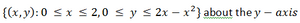# Volumes | Extension 2

1 comment

Edited: Jun 25, 2017

This question was presented by Johnny from our Ext. 2 maths class (2017) via wechat! : )

Use the method of slicing to find the volume of the solid obtained by rotating the region.tosophiechen
Jun 25, 2017Edited: Jun 25, 2017

Here is answered solution from Harry.# More challenging questions wanted!

New Posts
• ## Yr10 James Ruse Exam Question

Hi, I thought I would share this. Found this challenging at first until I studied polynomials further for yr 10 course. Found this question in one of the James Ruse year 10 papers on the net. Part i) was a little confusing at first, I didn't see it in any text books. Found it finally in book 2 of the HEC Yr 10 Polynomials where it mentions under Polynomial Division, that the degree of the Remainder is always less than the degree of the Divisor. In this case the Divisor is quadratic and of degree 2. So the Remainder is linear of degree 1. Answer: The remainder always has a lower degree than the divisor. Since the divisor is quadratic R(x)=ax+b which is a general form of a linear expression (ie of degree 1). Part ii) Simply use the remainder theorem and sub. in 4 for P(x) and R(x). Then find 4a+b as common to both. Took a pic of the solution. Part iii) Use part ii) relationship for a and b variables, apply Remainder Theorem P(-1)=5 since divisor is (x-(-1)) or (x+1) to get answer. See pic of solution.